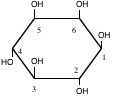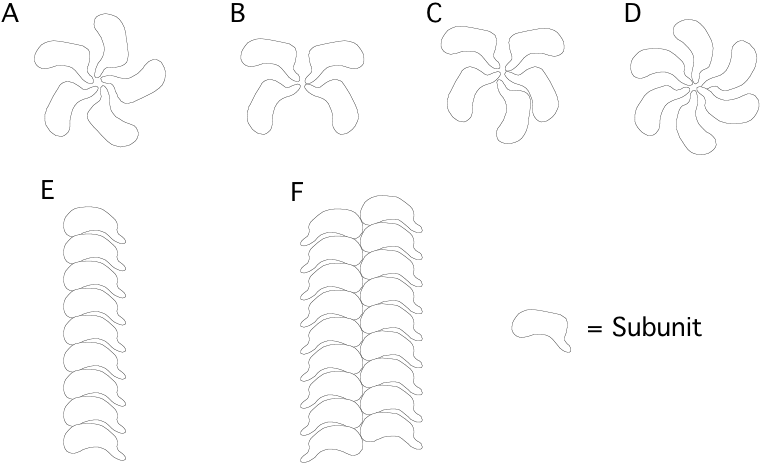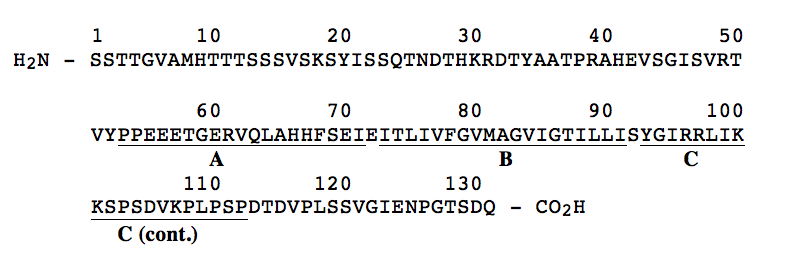# Sample Midterm 2

$$\newcommand{\vecs}{\overset { \rightharpoonup} {\mathbf{#1}} }$$ $$\newcommand{\vecd}{\overset{-\!-\!\rightharpoonup}{\vphantom{a}\smash {#1}}}$$$$\newcommand{\id}{\mathrm{id}}$$ $$\newcommand{\Span}{\mathrm{span}}$$ $$\newcommand{\kernel}{\mathrm{null}\,}$$ $$\newcommand{\range}{\mathrm{range}\,}$$ $$\newcommand{\RealPart}{\mathrm{Re}}$$ $$\newcommand{\ImaginaryPart}{\mathrm{Im}}$$ $$\newcommand{\Argument}{\mathrm{Arg}}$$ $$\newcommand{\norm}{\| #1 \|}$$ $$\newcommand{\inner}{\langle #1, #2 \rangle}$$ $$\newcommand{\Span}{\mathrm{span}}$$ $$\newcommand{\id}{\mathrm{id}}$$ $$\newcommand{\Span}{\mathrm{span}}$$ $$\newcommand{\kernel}{\mathrm{null}\,}$$ $$\newcommand{\range}{\mathrm{range}\,}$$ $$\newcommand{\RealPart}{\mathrm{Re}}$$ $$\newcommand{\ImaginaryPart}{\mathrm{Im}}$$ $$\newcommand{\Argument}{\mathrm{Arg}}$$ $$\newcommand{\norm}{\| #1 \|}$$ $$\newcommand{\inner}{\langle #1, #2 \rangle}$$ $$\newcommand{\Span}{\mathrm{span}}$$$$\newcommand{\AA}{\unicode[.8,0]{x212B}}$$

$\mathbf{BioSci\: 102\\ Sample\: Study\: Problems\: for\: Second\: Midterm}$

1. The aggregation of platelets in blood to form a plug on an injured surface of a blood vessel occurs after sequential activation of at least ten different enzymes which are normally present in blood as inactive precursors. Human platelets are composed of one major phospholipid, 1-stearoyl-2-arachidonyl phosphatidylinositol. The components of the phospholipid are stearic acid (18:0), arachidonic acid (20:4 all cis $$\Delta ^{5,8,11,14}$$), glycerol, phosphate, and inositol (structure shown below).Inositol

a) In the space below, draw the complete structure of the phospholipid 1-stearoyl-2-arachidonyl phosphatidylinositol, given that the linkage of inositol to the phosphatidate involves the hydroxyl group on carbon 1 of inositol. (SHOW ALL BONDS).

b) Sonication of the phospholipid described in part a) would most likely lead to the formation of (micelles, liposomes, two insoluble liquid phases). CIRCLE BEST ANSWER. Based on your answer, provide a diagrammatic description of the structures, if any, that would form upon sonication of this phospholipid. With your answer, please identify which of the components of the phospholipid will be exposed to the aqueous environment.

2. A region of a globular protein has the sequence GRAKSR.

a) Which of the following other regions of the same protein would be most likely to participate in a salt bridge with the above region? Give the reason for your answer.

A) WLIFTV

B) NEQKRL

C) NEQDHD

D) VILALL

Reason?-

b) Which two of the regions in a) could be held together tightly in the internal part of the protein by hydrophobic interactions? Give a reason for your answer.

Reason?

3. Name and draw the structural formulas for three amino acids that are not commonly found in an $$\alpha$$-helix and give a reason why each is rarely found in this configuration.

4. Identify three types of secondary structure commonly found in globular proteins:

1.

2.

3.

5. Much of the behavior of hemoglobin arises from the presence of salt bridges in the deoxy (tense) form. One of the main salt bridges is that between the histidine imidazole group of His 146 and the carboxylate group of Asp 94 (both on a single $$\beta$$ chain).

a) Draw this salt bridge (structural formulas) as it might exist in the tense form.

b) If a mutant hemoglobin appeared with a lysine replacing the histidine at position 146 of the $$\beta$$ chain, what effect would it have on the following (assume a pH of 7.0 if it is not otherwise given)? Explain the reason behind your answers.

1) The strength of the salt bridge.

2) The oxygen binding curve of Hb ($$\theta$$ versus PO2).

3) The Bohr effect in a shift from pH 7.0 to pH 7.2.

6. The acid-base properties of oxy- and deoxy-hemoglobin from a particular mammal can be described by the following two equilibrium expressions:

$\mathrm{Oxyhemoglobin\: HHbO_{2}\Leftrightarrow H^{+}+HbO_{2}\: pK_{a}=6.2}$

$\mathrm{Deoxyhemoglobin\: HHb\Leftrightarrow H^{+}+Hb\: pK_{a}=7.7}$

a) For each statement below place the letter "D" in the space provided if the statement is true of Deoxyhemoglobin, and the letter "O" if the statement is true of Oxyhemoglobin, or "D and O" if the statement is true for both proteins.

This form of Hb exhibits quaternary structure.

This form of Hb is a stronger acid than the other form.

At pH 6.5 this form of Hb binds more protons (mol H+/mole Hb) than the other form of Hb.

This form of Hb has a lower affinity for 2,3 - bisphosphoglycerate (BPG) than the other form.

As the $$\mathrm{P_{O_{2}}}$$ level is increased and the pH is kept constant, the concentration of this form of Hb will decrease.

b) A BIS102 student prepared 1 L of a 2 mM solution of the hemoglobin described above at pH 7.4 and measured its oxygen saturation to be 80%. Calculate the concentration of all of the forms of hemoglobin (i. e. $$\mathrm{Hb}$$, $$\mathrm{HHb}$$, $$\mathrm{HbO_{2}}$$, $$\mathrm{HHbO_{2}}$$) which will be found under these conditions.

c) The degree of oxygen saturation of hemoglobin can be used to monitor the production or consumption of $$\mathrm{H^{+}}$$ ions by a metabolic reaction. A change of oxygen saturation of the 0.002 M hemoglobin solution described in part "b" from 80% to 50% saturation indicates the (production or consumption) of $$\mathrm{H^{+}}$$ ions by a metabolic reaction taking place in the same tube. (Circle best answer). Assume a constant $$\mathrm{P_{O_{2}}}$$ level. Explain.

d) Calculate the number of moles of $$\mathrm{O_{2}}$$ released by the 1 L of hemoglobin solution described in part "c" when the oxygen saturation is changed from 80% to 50%. Provide a rationale for your answer based on the structure of hemoglobin.

7. Briefly describe the purpose of each of the following biochemical techniques (e. g. a method to separate carbohydrates on the basis of glucose content). Include a mention of a kind of biochemicals separated by the method. (3 pts. each)

a) Isoelectric focusing gel electrophoresis.

b) SDS-polyacrylamide gel electrophoresis.

c) Affinity chromatography.

d) Ion exchange chromatography.

e) Affinity labeling.

8. Which of the following are likely configurations of multimeric protein structures made up of identical subunits? [circle correct answer(s)]9. Shown below is the primary structure of a transmembrane protein component of a human red blood cell. Extensive antibody studies have shown that the first 52 amino acids (residues 1-52) of the protein lie on the exterior surface of the red blood cell while the carboxyl terminal 19 residues (113-131) were identified to lie on the inside surface of the red blood cell. On the structure shown below, the region between residues 53 and 112 has been divided into three peptide regions designated "A" (residues 53-72), "B" (residues 74-91) and "C" (residues 93-112). Of the three peptide regions, which region would most likely be found to extend through the lipid bilayer A, B, or C? CIRCLE BEST ANSWER.